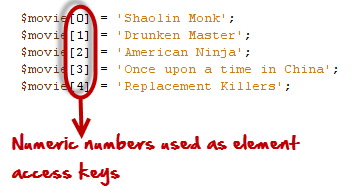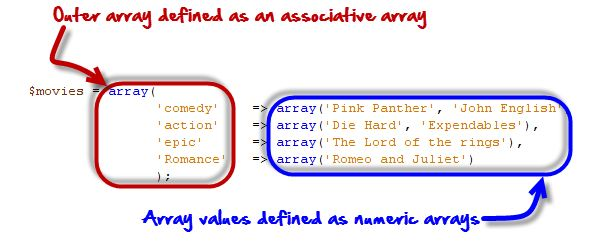# Arrays in PHP

## What is a PHP Array?

A PHP array is a variable that stores more than one piece of related data in a single variable.

Think of an array as a box of chocolates with slots inside.

The box represents the array itself while the spaces containing chocolates represent the values stored in the arrays.

The diagram below illustrates the above syntax.

In this tutorial, you will learn-

## Numeric Arrays

Numeric arrays use number as access keys.

An access key is a reference to a memory slot in an array variable.

The access key is used whenever we want to read or assign a new value an array element.

Below is the syntax for creating numeric array in php. Array Example

```<?php
\$variable_name[n] = value;
?>```

Or

```<?php
\$variable_name = array(n => value, …);
?>```

HERE,

• “\$variable_name…” is the name of the variable
• “[n]” is the access index number of the element
• “value” is the value assigned to the array element.

Let’s now look at an example of a numeric array.

Suppose we have 5 movies that we want to store in array variables.

We can use the example shown below to do that.

```<?php

\$movie = 'Shaolin Monk';
\$movie = 'Drunken Master';
\$movie = 'American Ninja';
\$movie = 'Once upon a time in China';
\$movie = 'Replacement Killers';

?>```

Here,Each movie is given an index number that is used to retrieve or modify its value. Observe the following code-

```<?php
\$movie="Shaolin Monk";
\$movie="Drunken Master";
\$movie="American Ninja";
\$movie="Once upon a time in China";
\$movie="Replacement Killers";
echo \$movie;
\$movie = " Eastern Condors";
echo \$movie;
?>```

Output:

`Once upon a time in China Eastern Condors`

As you can see from the above examples, working with arrays in PHP when dealing with multiple values of the same nature is very easy and flexible.

Alternatively, the above array variables can also be created using the following code.

```<?php
\$movie = array(0 => "Shaolin Monk",
1 => "Drunken Master",
2 => "American Ninja",
3 => "Once upon a time in China",
4 =>"Replacement Killers" );
echo \$movie;
?>```

Output:

`Replacement Killers`

## PHP Associative Array

Associative array differ from numeric array in the sense that associative arrays use descriptive names for id keys.

Below is the syntax for creating associative array in php.

```<?php
\$variable_name['key_name'] = value;

\$variable_name = array('keyname' => value);
?>```

HERE,

• “\$variable_name…” is the name of the variable
• “[‘key_name’]” is the access index number of the element
• “value” is the value assigned to the array element.

Let’s suppose that we have a group of persons, and we want to assign the gender of each person against their names.

We can use an associative array to do that.The code below helps us to do that.

```<?php
\$persons = array("Mary" => "Female", "John" => "Male", "Mirriam" => "Female");
print_r(\$persons);
echo "";
echo "Mary is a " . \$persons["Mary"];
?>```

HERE,Output:

`Array ( [Mary] => Female [John] => Male [Mirriam] => Female ) Mary is a Female`

Associative array are also very useful when retrieving data from the database.

The field names are used as id keys.

## PHP Multi-dimensional arrays

These are arrays that contain other nested arrays.

The advantage of multidimensional arrays is that they allow us to group related data together.

Let’s now look at a practical example that implements a php multidimensional array.

The table below shows a list of movies by category.

Movie titleCategory
Pink PantherComedy
John EnglishComedy
Die HardAction
ExpendablesAction
The Lord of the ringsEpic
Romeo and JulietRomance
See no evil hear no evilComedy

The above information can be represented as a multidimensional array. The code below shows the implementation.

```<?php
\$movies =array(
"comedy" => array("Pink Panther", "John English", "See no evil hear no evil"),
"action" => array("Die Hard", "Expendables"),
"epic" => array("The Lord of the rings"),
"Romance" => array("Romeo and Juliet")
);
print_r(\$movies);
?>```

HERE,Output:

`Array ( [comedy] => Array (  => Pink Panther  => John English  => See no evil hear no evil ) [action] => Array (  => Die Hard  => Expendables ) [epic] => Array (  => The Lord of the rings ) [Romance] => Array (  => Romeo and Juliet ) )`

Another way to define the same array is as follows

```<?php
\$film=array(

"comedy" => array(

0 => "Pink Panther",

1 => "john English",

2 => "See no evil hear no evil"

),

"action" => array (

0 => "Die Hard",

1 => "Expendables"

),

"epic" => array (

0 => "The Lord of the rings"

),

"Romance" => array

(

0 => "Romeo and Juliet"

)

);
echo \$film["comedy"];
?>```

Output:

`Pink Panther`

Note: the movies numeric array has been nested inside the categories associative array

## PHP Arrays: Operators

OperatorNameDescriptionHow to do itOutput
x + yUnionCombines elements from both arrays
```<?php
\$x = array('id' => 1);

\$y = array('value' => 10);

\$z = \$x + \$y;
?>```
Array([id] => 1 [value] => 10)
X == yEqualCompares two arrays if they are equal and returns true if yes.
```<?php
\$x = array("id" => 1);

\$y = array("id" => "1");

if(\$x == \$y)
{
echo "true";
}
else
{
echo "false";

}
?>```
True or 1
X === yIdenticalCompares both the values and data types
```<?php
\$x = array("id" => 1);

\$y = array("id" => "1");

if(\$x === \$y)
{
echo "true";
}
else
{
echo "false";
}
?>```
False or 0
X != y, x <> yNot equal
```<?php
\$x = array("id" => 1);

\$y = array("id" => "1");

if(\$x != \$y)
{
echo "true";
}
else
{
echo "false";
}
?>```
False or 0
X !== yNon identical
```<?php
\$x = array("id" => 1);

\$y = array("id" => "1");

if(\$x !== \$y)
{
echo "true";
}
else
{
echo "false";
}
?>```
True or 1

## PHP Array Functions

### Count function

The count function is used to count the number of elements that an php array contains. The code below shows the implementation.

```<?php
\$lecturers = array("Mr. Jones", "Mr. Banda", "Mrs. Smith");
echo count(\$lecturers);
?>```

Output:

`3`

### is_array function

The is_array function is used to determine if a variable is an array or not. Let’s now look at an example that implements the is_array functions.

```<?php
\$lecturers = array("Mr. Jones", "Mr. Banda", "Mrs. Smith");
echo is_array(\$lecturers);
?>```

Output:

`1`

### Sort

This function is used to sort arrays by the values.

If the values are alphanumeric, it sorts them in alphabetical order.

If the values are numeric, it sorts them in ascending order.

It removes the existing access keys and add new numeric keys.

The output of this function is a numeric array

```<?php
\$persons = array("Mary" => "Female", "John" => "Male", "Mirriam" => "Female");

sort(\$persons);

print_r(\$persons);
?>```

Output:

`Array (  => Female  => Female  => Male )`

### ksort

This function is used to sort the array using the key. The following example illustrates its usage.

```<?php
\$persons = array("Mary" => "Female", "John" => "Male", "Mirriam" => "Female");

ksort(\$persons);

print_r(\$persons);
?>```

Output:

`Array ( [John] => Male [Mary] => Female [Mirriam] => Female )`

### asort

This function is used to sort the array using the values. The following example illustrates its usage.

```<?php

\$persons = array("Mary" => "Female", "John" => "Male", "Mirriam" => "Female");

asort(\$persons);

print_r(\$persons);

?>```

Output:

`Array ( [Mary] => Female [Mirriam] => Female [John] => Male )`

### Why use arrays?

• Contents of Arrays can be stretched,
• Arrays easily help group related information such as server login details together
• Arrays help write cleaner code.

## Summary

• Arrays are special variables with the capacity to store multi values.
• Arrays are flexibility and can be easily stretched to accommodate more values
• Numeric arrays use numbers for the array keys
• PHP Associative array use descriptive names for array keys
• Multidimensional arrays contain other arrays inside them.
• The count function is used to get the number of items that have been stored in an array
• The is_array function is used to determine whether a variable is a valid array or not.
• Other array functions include sort, ksort, assort etc.

#### Top Courses